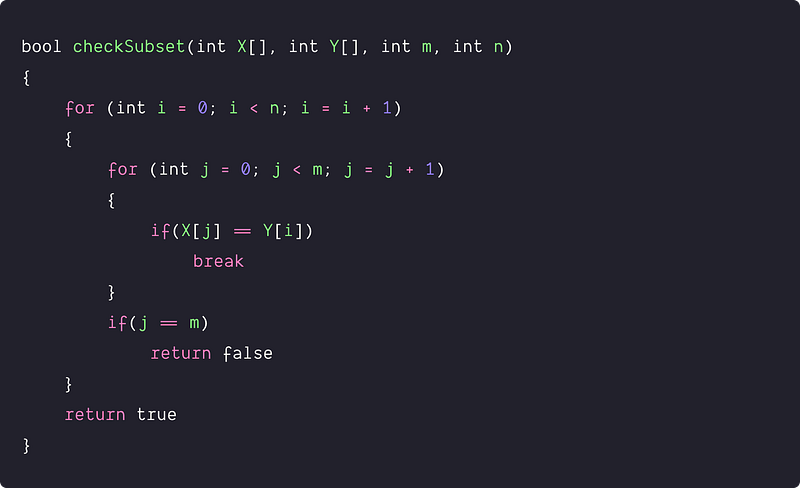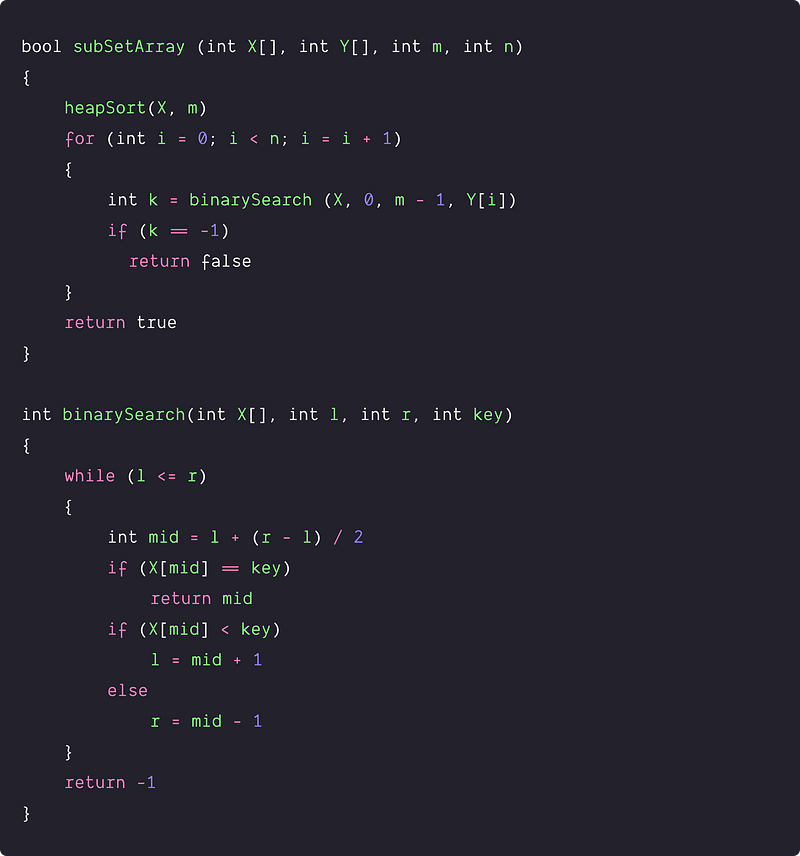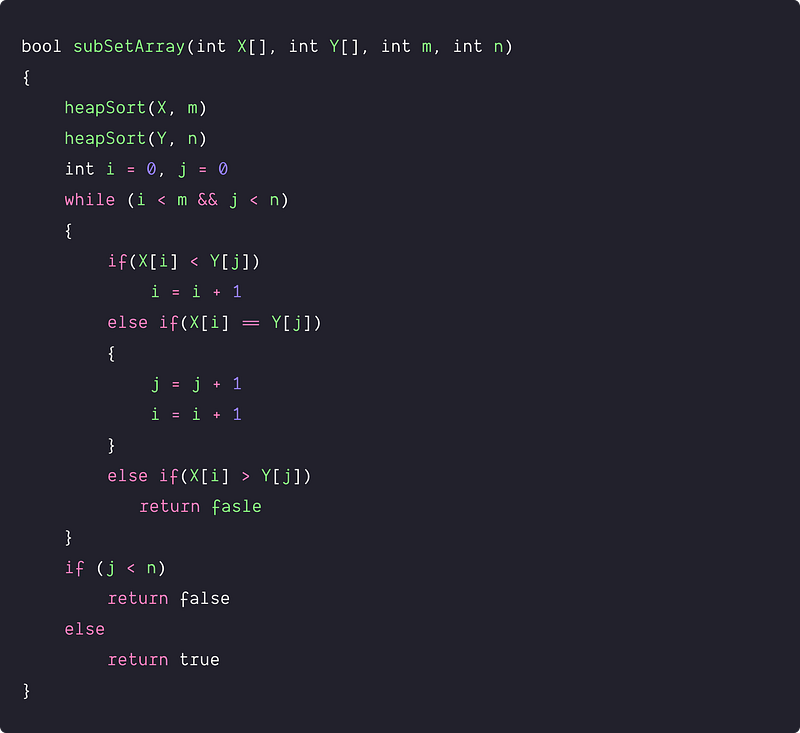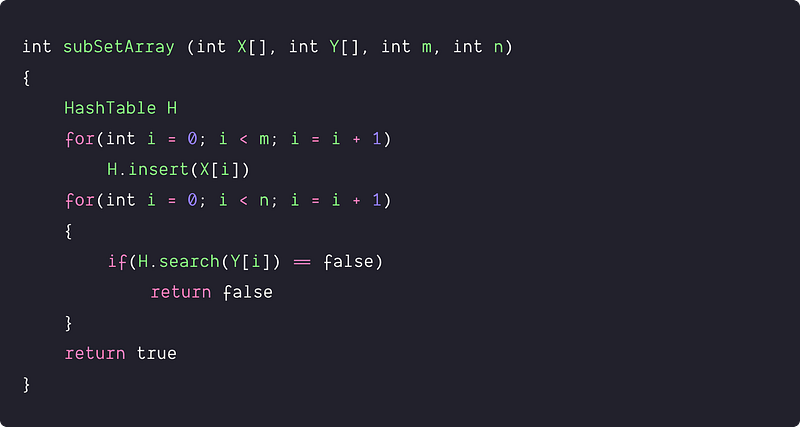# Find whether an array is a subset of another array

Key takeaway: An excellent-searching problem to learn time complexity optimization using various approaches. We continuously improve the searching time complexity to improve the overall time complexity of the solution. The two-pointers and hash table solution are intuitive and worth exploring.

### Let’s understand the problem

Given two unsorted arrays X[] and Y[] of size m and n, write a program to check whether Y[] is a subset of X[] or not.

• Y[] is a subset of X[] if each element of Y[] is present in X[].
• There are no repeated elements in both arrays.
• Assume that n <= m i.e. X[] is the larger array.

Example 1

Input: X[] = [2, 8, 12, 6, 10, 11], Y[] = [8, 2, 6, 11]

Output: True i.e. Y[] is a subset of X[]

Explanation: All the elements in array Y[] are present in array X[].

Example 2

Input: X[] = [6, 4, 8, 3], Y[] = [4, 7, 9]

Output: False i.e. Y[] is not subset of X[]

Explanation: Values 7 and 9 of array Y[] are not present in array X[].

### Discussed solution approaches

• A brute force approach  using two nested loops
• Using sorting and binary search
• Using sorting and two pointers approach
• An efficient approach  using a hash table

### Brute force approach  using two nested loops

Solution Idea

A simple idea would be to search each element of Y[] in X[] using linear search. If all elements of Y[] are present in X[], then Y[] is a subset of X[]. Otherwise, Y[] is not a subset of X[]. We use nested loops to implement this.

Solution PseudocodeTime and space complexity analysis

In the above-nested loops: the outer loop runs n times, and the inner loop is running m times. So worst-case time complexity would be O(m*n). But if we observe closely, the time complexity depends on the order of the elements in both arrays. It's an exercise for learners to think about the best and worst scenarios of the input. Explore!

We are using constant extra space, so space complexity = O(1)

### Using sorting and binary search

Now the critical question is: how can we improve the time complexity? Let's think!

Solution Idea

If we sort the larger array X[], then we can easily apply binary search to search each element of Y[] in X[]. Binary search performs searching in O(logn) on the sorted array, which can help to improve the time complexity further. Suppose we use one of the fastest sorting algorithms heap sort and iterative binary search for the implementation.

Solution Steps

• Sort the array X[] in increasing order using heap sort.
• We run a loop to search each element of Y[] in X[] using binary search. If any element Y[i] is not found in X[], then we return false. Otherwise, we move to the next iteration.
• By the end of the loop, all elements of Y[] are present in X[], and we return true.

Solution PseudocodeTime and space complexity analysis

Time Complexity = Time complexity of heap sort + n * time complexity of the iterative binary search = O(mlogm) + n. O(logm) = O(mlogm + nlogm)

Space Complexity = Space complexity of heap sort + Space complexity of the iterative binary search = O(1) + O(1) = O(1)

### Using sorting and two pointers approach

Solution Idea and Steps

Sometimes two pointers approach works perfectly on the sorted arrays. How do we apply it here to improve the time complexity? Here is an idea:

• Do the preprocessing by sorting both arrays.
• Apply a two-pointers approach similar to the merging algorithm. We can refer to the merging process of the merge sort algorithm.

How can we implement the above idea? Suppose we sort X[] and Y[] and initialize the two pointers i and j with starting elements of both arrays i.e. i =0 and j =0. Now we compare the elements one by one by running a loop till i < m && j < n.

• If(X[i] < Y[j]), then we have not yet found the value Y[j] in X[]. So we move the pointer i by 1 to search Y[j] in the remaining part of X[].
• If (X[i] == Y[j]), then we have found a value common to both arrays, which is part of the common subset. Now we move both pointers i and j by 1 to search the next value Y[j+1] in the remaining values of X[] (from index i + 1 to m -1). Think!
• if(X[i] > Y[j]), then remaining value in the X[] (from index i+ 1 to m-1) will be definitely greater than Y[j]. It means Y[j] is not present in X[]. In such a situation, we return false i.e. Y[] is not a subset of X[].
• Boundary condition: by the end of the loop, if(j < n), it means pointer j has not reached the end of the Y[]. So the remaining values in Y[] (from index j to n - 1) are not present in the X[]. So both arrays are not subset of each other, and we return false. Otherwise, if (j == n), then we have found each value of Y[] in X[], and we return true.

Solution PseudocodeTime and space complexity analysis

At each iteration of the loop, we compare one element each from X[] and Y[]. We increment the pointer i or j or both by 1, depending on the comparison. Total number of comparisons in the worst case = O(m + n), So time complexity = O(m + n). But if we observe closely, the time complexity depends on the order of the elements in both arrays. It's an exercise for learners to think about the best and worst scenarios of the input. Explore!

Suppose we are using heap sort for the implementation. Overall time complexity = Time complexity of sorting array X[] + Time Complexity of sorting array Y[] + Time complexity of two pointer approach of merging = O(mlogm) + O(nlogn) + O(m + n) = O(mlogm + nlogn)

Space Complexity = Space complexity of heap sort + space complexity of the merging loop = O(1) + O(1) = O(1)

### An efficient approach  using a hash table

Now critical question is: can we improve the time complexity to O(n)? Can we solve the problem without using sorting? Think!

Solution Idea

Here searching is an essential operation because we have to search for each value of Y[] in X[]. So we can improve the time complexity by using an efficient data structure for searching. The idea would be to use Hash Table to perform searching and insertion efficiently in O(1) average.

Solution Steps

• Take a Hash Table of size O(m)
• Insert all the elements of array X[] into the Hash Table
• Traverse Y[] and search for each element of Y[] in the Hash Table. If any element is not present in the hash table, then we return false.
• If we have elements are found all elements of Y[] in the hash table, then we return true.

Solution PseudocodeTime and space complexity analysis

Time complexity = Time complexity of inserting m elements of X[] in hash table + Time complexity of searching n elements of Y[] in hash table = m. O(1) + n . O(1) = O(m) + O(n) = O(m+n)

Space complexity = Extra space of Hash Table = O(m)

### Critical ideas to think!

• In the last approach, what would be the time and space complexity if we use a self-balancing BST in place of a hash table?
• What would be the worst and best case input in the brute force approach? Count the exact number of comparison operations in the worst-case scenario.
• In the 2nd approach, can we solve the problem by sorting the smaller array Y[]?
• Is all the above algorithm works fine if elements are repeated? Check and confirm this by tracking the algorithms via some examples?
• What would be the worst-case input in the two-pointers approach? Count the exact number of comparison operations in the worst-case scenario.
• Can we solve this problem using some other approach?
• What would be the time and space complexity if we use quick-sort or merge sort In the 2nd and 3rd approaches?
• Why the idea of the two-pointer approach works perfectly for a sorted array? Verify the correctness of the 3rd approach.

### Comparisons of time and space complexities

• Nested loops: Time = O(nm), Space = O(1)
• Sorting and binary search: Time = O(mlogm + nlogm), Space = O(1)
• Sorting and two pointers: Time = O(mlogm + nlogn), Space = O(1)
• Hash Table approach: Time = O(m + n), Space = O(m)

### Suggested coding problems to practice

Enjoy learning, Enjoy coding, Enjoy algorithms!

Subscribe to get well-designed content on data structures and algorithms, machine learning, system design, oops, and mathematics. enjoy learning!

### We Welcome Doubts and Feedback!